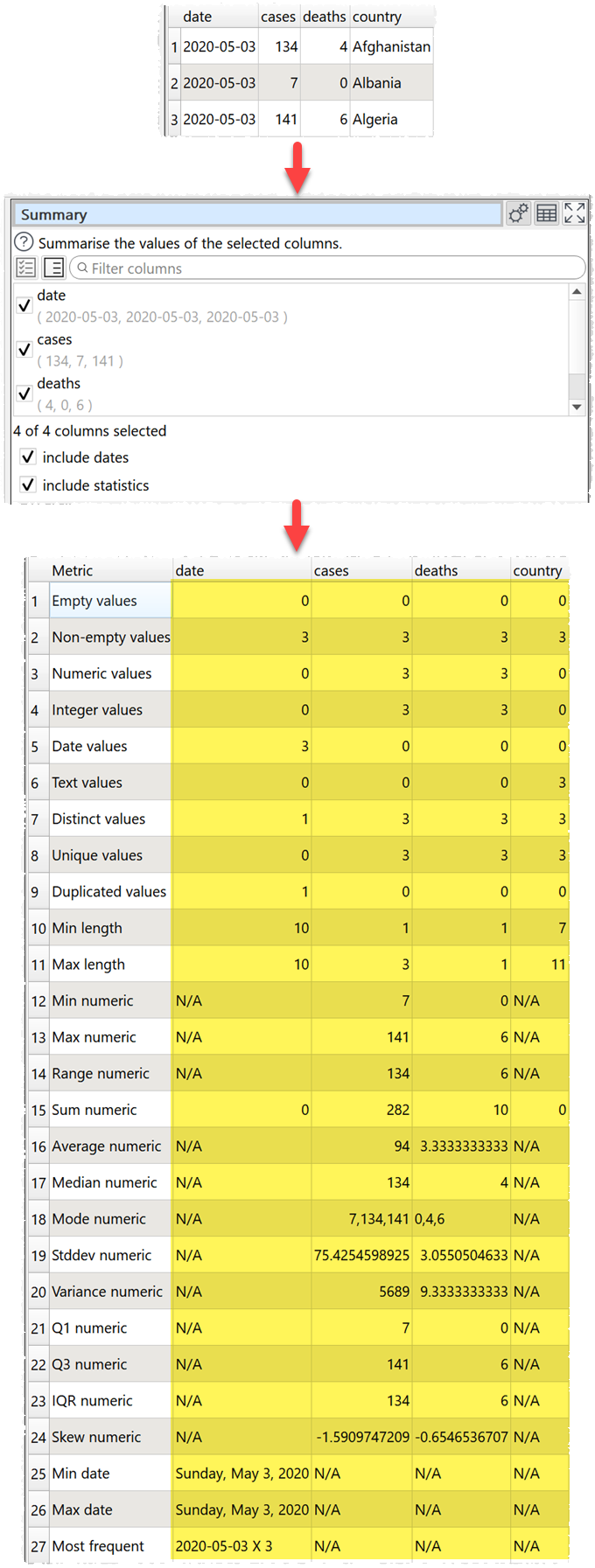﻿ Reference > Transforms > Summary

# Summary

## Description

Summarize the values in the selected columns.

## Example

Create a summary, including dates and statistics:One.

## Options

Select the Columns you wish to summarize.

Check include dates if you wish to check for date values using supported date formats. This can be slow for large datasets.

Check include statistics if you wish to add the sum, average, median and standard deviation for numeric values.

## Notes

Whether values are interpreted as number or dates depends on Supported date formats and Locale in the Preferences window.

Empty values is the number of values in the column that are completely empty. Values with whitespace do not count as empty.

Non-empty values is the number of values in the column that are not completely empty. Values with whitespace do not count as empty.

Numeric values is the number of numeric of values in the column that can be interpreted as a number.

Integer values is number of numeric of values in the column that can be interpreted as integers (whole numbers). "1.0" (depending on your Locale), "0", "-1" and "1e3" are considered integers.

Date values is the number of values in the column that can be interpreted as a date. Only shown if check for dates is checked.

Text values is the number of values in the column that cannot be interpreted as empty, numeric or date.

Distinct values is the number of different values in the column. Date and numeric values are treated as text (e.g. '7' is treated as different to '7.0' and '1/1/2020' is treated as different to '01/01/2020'). Comparison between values is sensitive to case and whitespace. Empty values are not counted.

Unique values is the number of values that only occur once in the column. Date and numeric values are treated as text. Comparison between values is sensitive to case and whitespace. Empty values are not counted.

Duplicated values is the number of values that only more than once in the column. Date and numeric values are treated as text. Comparison between values is sensitive to case and whitespace. Empty values are not counted.

Min length is the minimum number of characters of a value in the column. Whitespace is counted. Date and numeric values are treated as text.

Max length is the maximum number of characters of a value in the column. Whitespace is counted. Date and numeric values are treated as text.

Min numeric is the minimum numeric value in the column. Non-numeric and empty values are ignored.

Max numeric is the maximum numeric value in the column. Non-numeric and empty values are ignored.

Range numeric is Max numeric - Min numeric.

Sum numeric is the sum of numeric values in the column. Non-numeric and empty values are ignored. Only shown if include statistics is checked.

Average numeric is the arithmetic mean of numeric values in the column. Non-numeric and empty values are ignored. Only shown if include statistics is checked.

Median numeric is the median of numeric values in the column. Non-numeric and empty values are ignored. Only shown if include statistics is checked.

Mode numeric is the mode of numeric values in the column. Non-numeric and empty values are ignored. Only shown if include statistics is checked.

Stddev numeric is the sample standard deviation (equivalent to Excel function stddev.s) of numeric values in the column. Non-numeric and empty values are ignored. Only shown if include statistics is checked.

Variance numeric is the square of the sample standard deviation. Only shown if include statistics is checked.

Q1 numeric is the first quartile (calculated using Method 1 here). Only shown if include statistics is checked.

Q3 numeric is the third quartile  (calculated using Method 1 here). Only shown if include statistics is checked.

IQR numeric is the Inter Quartile Range. Only shown if include statistics is checked.

Skew numeric is the alternative Pearson Mode Skewness  (3 * (Mean – Median) / Standard Deviation ). Only shown if include statistics is checked.

Min date is the minimum date value in the column. Only shown if include dates is checked.

Max date is the maximum date value in the column. Only shown if include dates is checked.

Most frequent lists the most common text in the column. Empty values are not counted. Date and numeric values are treated as text. Comparison between values is sensitive to case and whitespace.

## Notes

Mode may not work as expected with non-integer values, due to precision issues. You can fix this by using a Num Format transform first.

Use Num Format to change the precision of numerical results.

You can use Whitespace to remove any whitespace at the start or end of values before Summary.

If you wish to have a row displayed per column you can Transpose the table first.